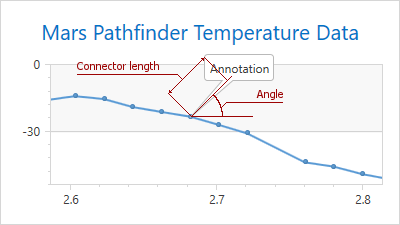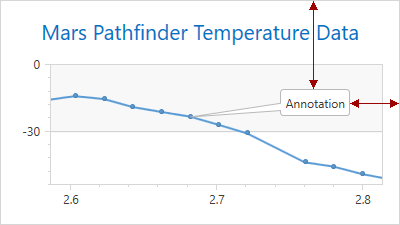21.2
21.1
20.2
20.1
19.2
19.1
18.2
18.1
17.2

# Annotation.ShapePosition Property

Specifies the annotation position.

Namespace: DevExpress.Xpf.Charts

Assembly: DevExpress.Xpf.Charts.v21.2.dll

## Declaration

``public AnnotationShapePosition ShapePosition { get; set; }``

## Property Value

Type Description
AnnotationShapePosition

An AnnotationShapePosition class descendant representing the shape position type.

## Remarks

The ShapePosition property can be specified as the RelativePosition or FreePosition type.

The following table uses examples to describe these two annotation shape position types.

The Property Values

The Example Image

Description

ShapePosition = RelativePositionIf the Annotation.ShapePosition property is specified as the RelativePosition type, use the RelativePosition.ConnectorLength and RelativePosition.Angle properties to modify the annotation shape position.

In this example, the ConnectorLength property is set to 70 and the RelativePosition.Angle property is 45.

ShapePosition = FreePositionIf the Annotation.ShapePosition property is specified as the FreePosition type, you can use the FreePosition.VerticalAlignment and FreePosition.HorizontalAlignment properties to specify the annotation layout. To define the parent element, use the FreePosition.DockTarget property. To define the indents from the parent element edges, use the Annotation.Margin property.

In this example, the annotation is docked to a Chart control. The HorizontalAlignment property is set to Right and the VerticalAlignment property is set to Top. The Margin property is defined as (0, 90, 50, 0).

## Examples

### Add an Annotation in Markup

This example demonstrates how to anchor an annotation to a series point.

To do this, specify the SeriesPointAnchorPoint.SeriesPoint property.

``````<dxc:Annotation Content="Annotation #1">
<dxc:Annotation.AnchorPoint>
<dxc:SeriesPointAnchorPoint SeriesPoint="{Binding ElementName=seriesPoint}"/>
</dxc:Annotation.AnchorPoint>
<dxc:Annotation.ShapePosition>
<dxc:RelativePosition Angle="60"
ConnectorLength="50"/>
</dxc:Annotation.ShapePosition>
</dxc:Annotation>
``````

### Add an Annotation in Code

The following example creates an annotation that is anchored to a pane.

``````<dxc:ChartControl x:Name="chartControl">
<dxc:XYDiagram2D x:Name="diagram">
<dxc:XYDiagram2D.SecondaryAxesX>
<dxc:SecondaryAxisX2D x:Name="xAxis"/>
</dxc:XYDiagram2D.SecondaryAxesX>
<dxc:XYDiagram2D.SecondaryAxesY>
<dxc:SecondaryAxisY2D x:Name="yAxis"/>
</dxc:XYDiagram2D.SecondaryAxesY>
<dxc:XYDiagram2D.Panes>
<dxc:Pane x:Name="pane"/>
</dxc:XYDiagram2D.Panes>
<!--...-->
</dxc:XYDiagram2D>
</dxc:ChartControl>
``````
``````using DevExpress.Xpf.Charts;
//...
private void Window_Loaded(object sender, RoutedEventArgs e) {
Annotation annotation = new Annotation();
annotation.Content = "Annotation";
annotation.AnchorPoint = new PaneAnchorPoint {
AxisXCoordinate = new AxisXCoordinate { AxisValue = new TimeSpan(0, 0, 5), Axis = xAxis },
AxisYCoordinate = new AxisYCoordinate { AxisValue = 100, Axis = yAxis },
Pane = pane
};
annotation.ShapePosition = new RelativePosition { Angle = 30, ConnectorLength = 60 };
}
``````

If the chart is bound to data and you want to anchor an annotation to a series point, use the ChartControl.BoundDataChanged event to access the point collection. The following example creates an annotation for each point of a spline series.

``````<dxc:ChartControl x:Name="chartControl"
BoundDataChanged="chartControl_BoundDataChanged">
<dxc:XYDiagram2D x:Name="diagram">
<dxc:SplineSeries2D>
<!--...-->
</dxc:SplineSeries2D>
</dxc:XYDiagram2D>
</dxc:ChartControl>
``````
``````using DevExpress.Xpf.Charts;
//...
private void chartControl_BoundDataChanged(object sender, RoutedEventArgs e) {
SplineSeries2D series = (SplineSeries2D)diagram.Series;
if (series.Points.Count > 0) {
for (int i = 0; i < series.Points.Count; i++) {
chartControl.Annotations.BeginInit();
Annotation annotation = new Annotation() {
Content = series.Points[i].Value.ToString()
};
annotation.AnchorPoint = new SeriesPointAnchorPoint() {
SeriesPoint = series.Points[i]
};
annotation.ShapePosition = new RelativePosition() {
Angle = 0,
ConnectorLength = 0
};